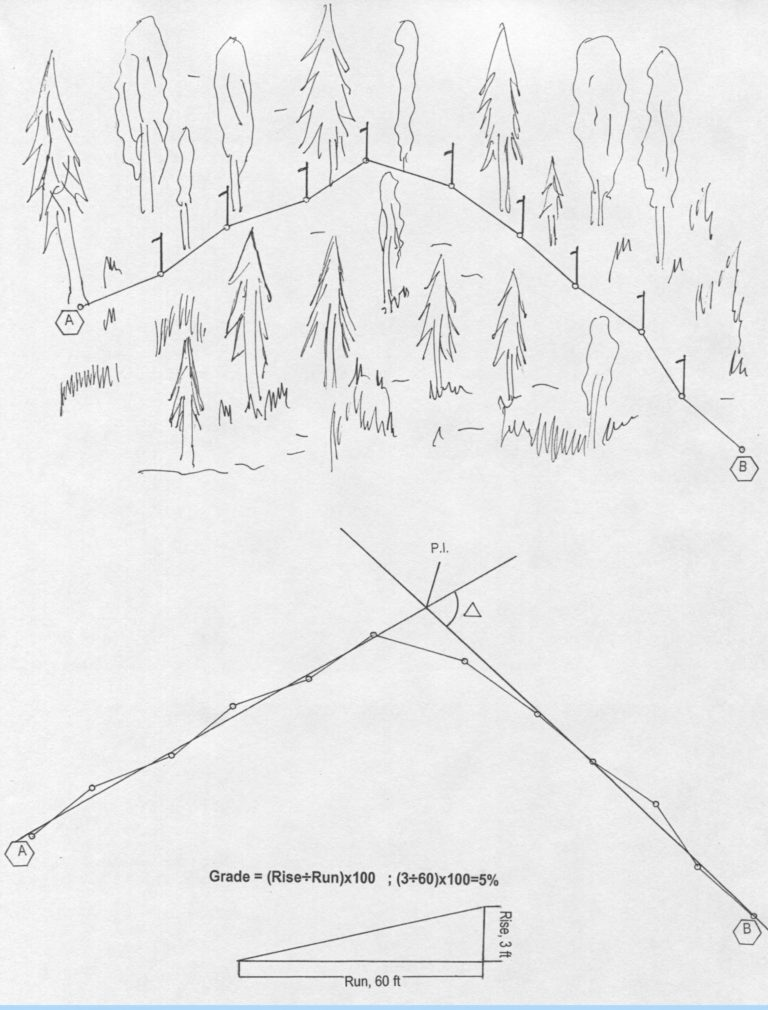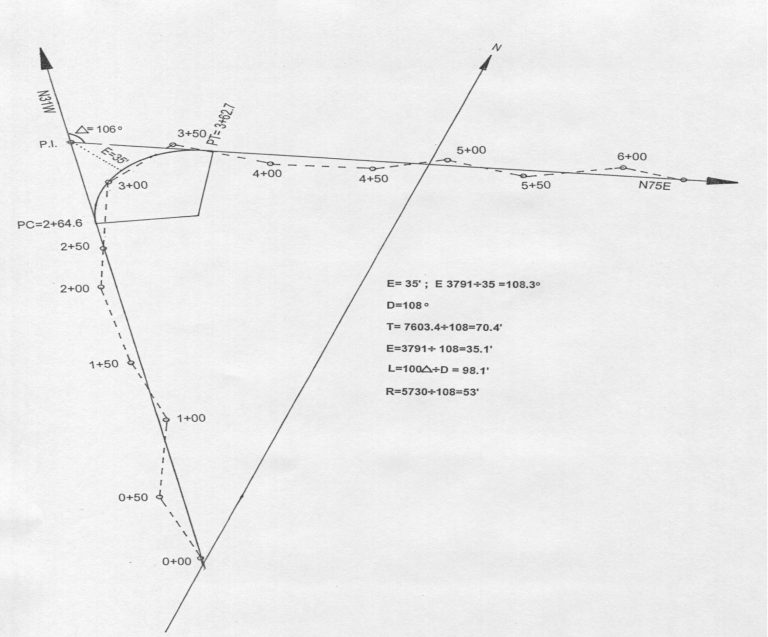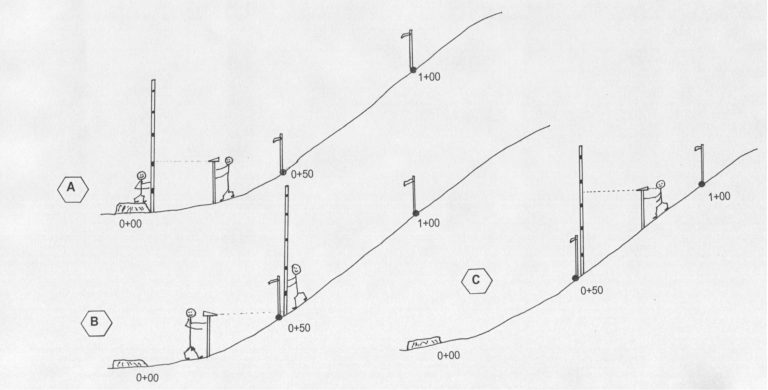# Basics of Forest Road Surveying

## Basics of Forest Road Surveying

Development of sound forest roads is one of the major parameters influencing overall timber harvesting activities within the perspective of sustainable forestry. This fact sheet summarizes some of the main aspects of forest road surveying, establishing curves and determining overall road length and profile of the road.Figure 1. Schematic of gradeline in the field.

Delta angle for each curve is calculated in the next step. Required parameters for each curve, namely external (E), point of curvature (PC) and point of tangency (PT) are calculated to determine geometry of each curve. External is the distance between PI and the closest point of the curve, and it is determined by measuring in the field. Derived from delta angle and E value, degree of the curve, which is defined by the angle subtended by 100 feet in the curve, is calculated based on table values. Figure 2 illustrates an example of establishing the centerline and calculations of curve parameters. In this example, the total length of the road will be determined by measuring from the first station to PC plus the length of the curve and distance from PT to the end of the road, which is the last station. When all measurements are obtained in the field, they are added together, and the total length of the road is determined. Also, data gathered in the field are transferred to engineering paper in the form of a scaled drawing so the parameters of the road can simply be quantified.

Table 1. Example for road profile. [HI=E+BS; E=HI-FS]

Station Backsight Reading (BS) Height of Instrument (HI) Foresight (FS) Elevation (E)
0+00 - - - 1,000
5.00 1,005 - -
0+50 - - 1.0 1,004
8.00 1,012 - -
1+00 - - 0.5 1,001.5
7.00 1,018.5 - -
1+50 - - 3.00 1,015.5Figure 2. Establishment of centerline and calculations of parameters of the curves.Figure 3.  Process of collecting data for the road profile.

Salim Hiziroglu